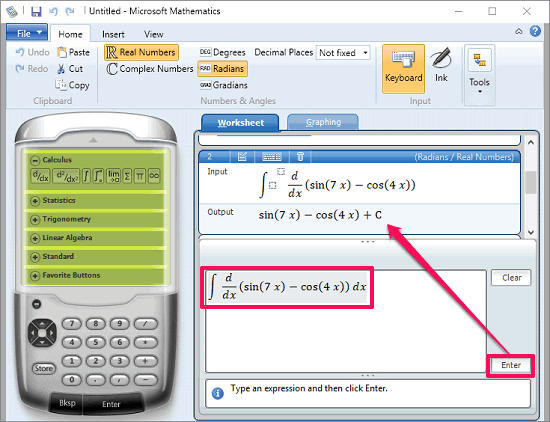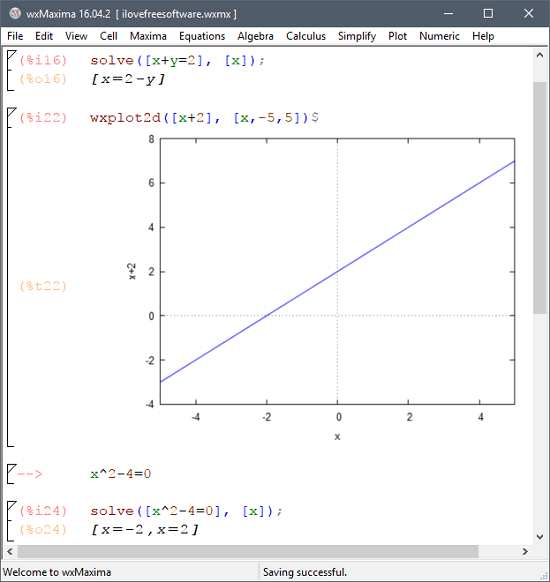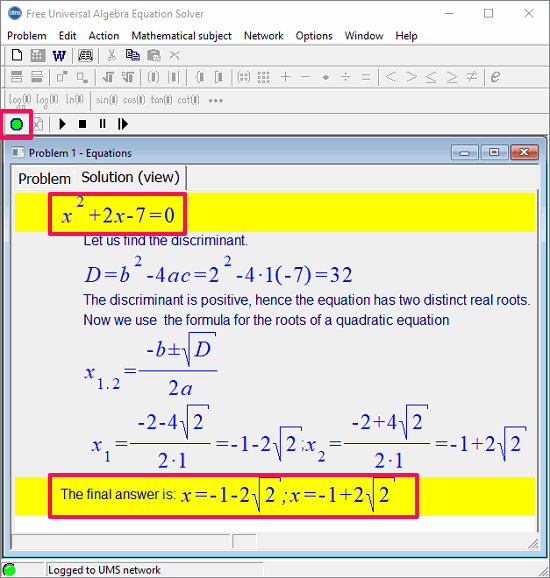Editor Ratings:
User Ratings:
[Total: 0 Average: 0]

Here are 4 free Mathematical equation solver software for Windows. These software help you enter equations, both simple algebraic and complex ones to solve them. These software take the equation entered by you and produce the corresponding result. Some of these software are quite smart and they offer nice features such as step by step solution of an equation, exporting the result to DOC file, etc.

These equation solver software can be a great help to high school students and teachers. Some of these software come with a very interactive interface that makes learning interesting. Also, some of these software come with support of plotting 2D and 3D graphs.Although the mathematical equations can be solved by using scientific calculators, but you may have noticed that they don’t allow you to enter the equation in standard form. That’s where these software come in handy. These software takes the equation that you manually write on the paper; they can understand it, and also solve it. So, now let’s see what are some best free mathematical equation solver software for Windows out there.

## Microsoft Mathematics

Microsoft Mathematics is one of the best free mathematical equation solver software for Windows. The software is very powerful to solve any equation whether it is linear, algebraic, quadratic, differential, or calculus. Microsoft Mathematics can solve them all and can plot the corresponding graph very easily. Apart from solving equations, the software comes with some other features as well, such as solving matrices, triangle, and unit converter. The Software comes with customizable interface and you can export the calculation result as MS Word or GCW file to any directory.The interface of Microsoft Mathematics is similar to other Microsoft applications, such as MS Word. And all the necessary options can be found in the upper ribbon of the software. First, grab the setup file of Microsoft Mathematics from the link above and then use it. The software comes with a built-in calculator that you can use for intermediary calculations. The software can easily handle system of equations or a single equation very easily. Just enter you equation in it and then hit the Enter button. After that, it will produce the corresponding output for you. See the above screenshot.

## Maxima

Maxima is a very powerful mathematical equation solver software for Windows. The software allows you to solve a lot of mathematical equations including algebraic, polynomial, linear, integral, differential, and lot more. You can type any equation on its interface and it will automatically recognize it and will solve it. Though, the software is quite large in size but it is not a problem as it offers a lot of features in it.

Apart from solving various types of equations, Maxima also lets you plot their equivalent 2D and 3D graphs. It also supports most of the common matrix related formulas and expressions in it.## Free Universal Algebra Solver

Free Universal Algebra Solver is a free version of its major software that offers solving linear and algebraic equations. The software is quite intelligent and solves an equation in step by step animation. You can also change the animation speed or if you want to just get the solution, then you can also do that. To get the solution for a particular linear or quadratic equation, you just have to enter it in its editor. After that, it will solve the equation and you can see the simulation on its solver window.It’s pretty easy to get started with free equation solver software. Just download and install it from above link and then launch it. After that, you will see the equation editor window where you have to type your desired equation. You can use the various tools from the toolbar such as log, natural log, fractions, subscript, matrix, etc.

When you have typed your equation in the editor, hit the green button to start solving it. After that, the software will start solving the entered equation step by step. If you want to see the solution in the simulation mode then you can wait for it to solve the whole answer. And if you just want to see the whole answer in a single shot, then hit the stop button and it will give you the answer. You can see the above screenshot.

Quadratic Equation Solver is another free equation solver software for Windows. But, this software can solve quadratic equations only. The software takes the coefficients as an input and then calculates the corresponding output. It calculates the solutions of an equation and show it to you. It can also indicate the validity of the roots of an equation that you enter in it. And if you want to verify your answer, then you can do that.It’s pretty easy to solve a quadratic equation using Quadratic Equation Solver. Just grab its portable executable file from above link and then lunch it. After that, you will see its interface where you can enter the coefficients of the quadratic equation that you want to solve. After that, hit the Answer button and it will calculate the roots of the quadratic equation. And after getting result, you can either close the software or start solving other quadratic equations.

## Final Words

These were some pretty awesome equation solver software for Windows. Using these software, you can easily solve various type of equations including quadratic, linear, algebraic, trigonometric, etc. And some of the above software come with other features as well, such as simulating the solution step by step, exporting to Word, unit converter, and some others. So, if you are looking for decent equation solver software, then these software will help you.

 Editor Ratings: User Ratings:[Total: 0 Average: 0] Tags: equationmathematics